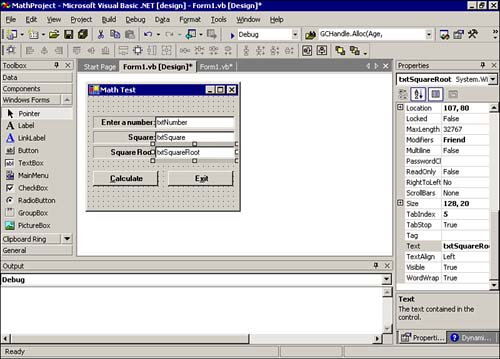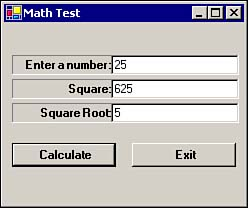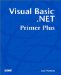# A Simple Math Program

In this section you'll write a simple program, the Math program, that exercises some numeric data. Although the program is quite simple, it illustrates some common tasks that you must consider when working with numeric data.

Start a new project and add three labels and three text boxes, as shown in Figure 4.9. Name the top text box txtNumber , name the second text box txtSquare , and name the last text box txtSquareRoot . You should name the Calculate button btnCalc and the Exit button btnExit . Notice that these names follow the naming conventions mentioned in Chapter 2.

##### Figure 4.9. The Math program form.You can leave the label names unchanged because you will not reference them in the program. However, the labels will look best if you set their properties as follows :

Property

Setting

BorderStyle

Fixed3D

Font

Microsoft Sans Serif

Font Style

Bold

TextAlign

MiddleRight

You should also modify the Text property for each label to correspond to the text shown in Figure 4.9.

Notice that the Text property for each of the buttons has an ampersand ( & ) embedded in it. For example, the Text property for the btnCalc button is &Calculate , and the btnExit button has the Text property set to E&xit . Using the ampersand like this means the program's user can use the Alt+C and Alt+X key combinations to activate the buttons instead of clicking the buttons with a mouse.

The only code you need to add to the program is that for the Calculate button. Add the following code to the btnCalc object's Click() event:

` Dim Square As Double, SquareRoot As Double  Square = CDbl(txtNumber.Text) txtSquare.Text = CStr(Square * Square) txtSquareRoot.Text = CStr(Sqrt(Square)) `

The first line simply creates two variables named Square and SquareRoot , using the Dim statement discussed earlier in this chapter.

#### Data Type Conversions

Visual Basic .NET does not like to fit square pegs into round holes. That is, Visual Basic .NET does not like to assign a text variable into a numeric variable. Therefore, you need to use a conversion routine when you try to perform assignments between different data types.

The second line:

` Square = CDbl(txtNumber.Text) `

converts the number that the user types into the txtNumber text box into a Double data type by using the built-in Visual Basic .NET CDbl keyword. (If you look at the table of keywords earlier in this chapter, in the section "Keywords," you should notice that CDbl is a Visual Basic .NET keyword.)

Why do you have to use the CDbl function? You use this function because the data in the txtNumber.Text text box is String data, not numeric data. The purpose of the CDbl function is to convert the String data to Double data. The value returned from the CDbl function is a numeric value of the Double data type. For example, suppose you pressed the 2 and the 5 keys, resulting in the character string "25" being placed in the txtNumber text box. These two ASCII characters correspond to the decimal values 50 (the 2 character) and 53 (the 5 character), as you can see in Appendix A. The two values 50 and 53 are stored in memory as String data types. Because you cannot perform mathematical operations directly on String data, you use the CDbl function to convert the string "25" to a Double data type with the numeric value 25 .

Therefore, this line:

` Square = CDbl(txtNumber.Text) `

becomes this:

` Square = CDbl("25") `

which then becomes this:

` Square = 25.0 `

This line assigns the numeric value 25.0 to the variable named Square .

The next line in the btnCalc object's Click() event is this:

` txtSquare.Text = CStr(Square * Square) `

This line does almost the opposite action of the previous line. In this case, Square holds a numeric value, but you want to assign the product of Square multiplied by itself to a text box. The problem is that Square is a numeric Double data type, but txtSquare.Text demands a String data type. As you might have already guessed, CStr is a Visual Basic .NET built-in function that converts numeric data into String data. Therefore, this line:

` txtSquare.Text = CStr(Square * Square) `

first multiplies Square by itself (the Square * Square expression) and then converts the result of that operation into a String data type. The String data returned by CStr can then be assigned directly to txtSquare.Text .

Now let's look at the last line:

` txtSquareRoot.Text = CStr(Sqrt(Square)) `

This line looks a little confusing, but it's actually pretty simple. Remember that expressions inside parentheses are resolved before anything else in the expression. Therefore, it appears that a function named Sqrt is called using the numeric value of Square as an argument. However, if you look at the list of keywords in the section "Keywords," earlier in this chapter, you can't find Sqrt . So what is Sqrt ? The answer is found in something called a library.

#### Visual Basic .NET Libraries

Sqrt is actually a math function that returns the square root of a number. However, the Sqrt function is not an inherent part of Visual Basic .NET. Instead, the Sqrt function is a routine found in the math library that comes with Visual Basic .NET. The math library is not automatically included in every program simply because not every program needs the functionality provided by the math library.

However, when you need some functionality from the math library, as you do here, you can tell Visual Basic .NET to include the math library in the program. You do this by adding the following line to the very top of your program:

` Imports System.Math `

This line tells Visual Basic .NET to include the math routines in the program. Doing this gives you access to the Sqrt function and other functions. In your program, if Square * Square is 25 , the Sqrt function returns the Double numeric value 5 . However, because this is a Double data type, you must again use the CStr function to convert the Double data type into a String data type so you can assign the result into txtSquareRoot.Text .

The only other code you need to add is the line:

` Me.Dispose() `

You add this line to the btnExit object's Click() event. Remember that Me is shorthand notation that always refers to the currently active form. The Dispose() method simply ends the program if the user clicks the btnExit button.

Figure 4.10 shows what the program output should look like if the user enters 25 in the txtNumber text box.

##### Figure 4.10. A sample run of the Math program.The Math program shows you how to convert from numeric to string data and string to numeric. Indeed, if you look at the keywords that begin with c, you can see that a number of built-in functions are provided for converting from one data type to another. You can use Visual Basic .NET's help system to get additional details on how to use these conversion routines.Visual Basic .NET Primer Plus
ISBN: 0672324857
EAN: 2147483647
Year: 2003
Pages: 238
Authors: Jack Purdum

Similar book on Amazon